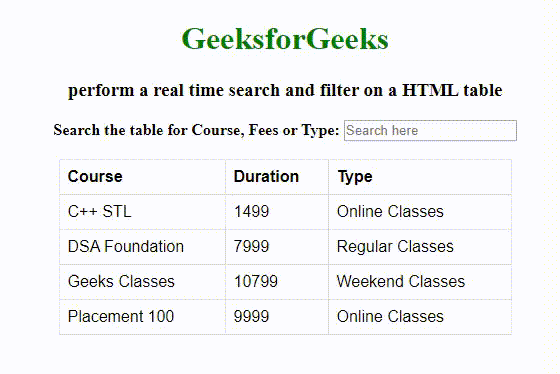GeeksforGeeks App
Open AppBrowser
Continue

# How to perform a real time search and filter on a HTML table?

Here, we learn how to perform a real-time search and filter on an HTML table. When a word is entered, all rows of the table will be searched (except the table head) and the rows containing the matching word will be displayed. For this, we can use JQuery methods.

• filter(): This method is used to filter out all the elements that do not match the selected criteria and those matches will be returned. Reduce the set of matched elements to those that match the selector or pass the function’s test.
• toggle(): This method is used to check the visibility of selected elements to toggle between hide() and show() for the selected elements. Display or hide the matched elements.

In the below example, the search value entered in the search box is stored in the variable named “value” and converted to lowercase since we are doing case insensitive search. After that, we search each row in the table using the filter() function and display the row where the string stored in variable “value” is found. The toggle() method is used to show the rows containing the search word and hide others. The indexOf(value) returns -1 if the word is not found in the row.

Example: Below example illustrates the use of filter() and toggle() function to perform a realtime search and filter on HTML table.

 ```<``html``>`` ` `<``head``>``    ``<``script` `src``=``"https://ajax.googleapis.com/ajax/libs/jquery/3.4.1/jquery.min.js"``>``    ```` ` `    ``<``style``>``        ``table {``            ``font-family: arial, sans-serif;``            ``border-collapse: collapse;``            ``width: 50%;``        ``}``         ` `        ``td,``        ``th {``            ``border: 1px solid #dddddd;``            ``text-align: left;``            ``padding: 8px;``        ``}``         ` `        ``h1 {``            ``color: green;``        ``}``    `````` ` `<``body``>``    ``<``center``>``        ``<``h1``>GeeksforGeeks``        ``<``h3``>``          ``perform a real time search and filter ``          ``on a HTML table``        ````        ``<``b``>Search the table for Course, Fees or Type: ``          ``<``input` `id``=``"gfg"` `type``=``"text"` `                 ``placeholder``=``"Search here"``>``        ````        ``<``br``>``        ``<``br``>``        ``<``table``>``            ``<``tr``>``                ``<``th``>Course``                ``<``th``>Duration``                ``<``th``>Type``            ````            ``<``tbody` `id``=``"geeks"``>``                ``<``tr``>``                    ``<``td``>C++ STL``                    ``<``td``>1499``                    ``<``td``>Online Classes``                    ````                ````                ``<``tr``>``                    ``<``td``>DSA Foundation``                    ``<``td``>7999``                    ``<``td``>Regular Classes``                ````                ``<``tr``>``                    ``<``td``>Geeks Classes``                    ``<``td``>10799``                    ``<``td``>Weekend Classes``                ````                ``<``tr``>``                    ``<``td``>Placement 100``                    ``<``td``>9999``                    ``<``td``>Online Classes``                ````            ````        ```` ` `        ``<``script``>``            ``\$(document).ready(function() {``                ``\$("#gfg").on("keyup", function() {``                    ``var value = \$(this).val().toLowerCase();``                    ``\$("#geeks tr").filter(function() {``                        ``\$(this).toggle(\$(this).text()``                        ``.toLowerCase().indexOf(value) > -1)``                    ``});``                ``});``            ``});``        ````  ```` ` ``` ` ``

Output:My Personal Notes arrow_drop_up
Related Tutorials